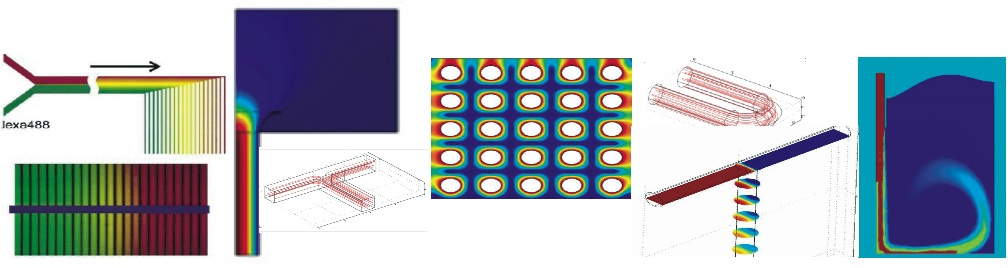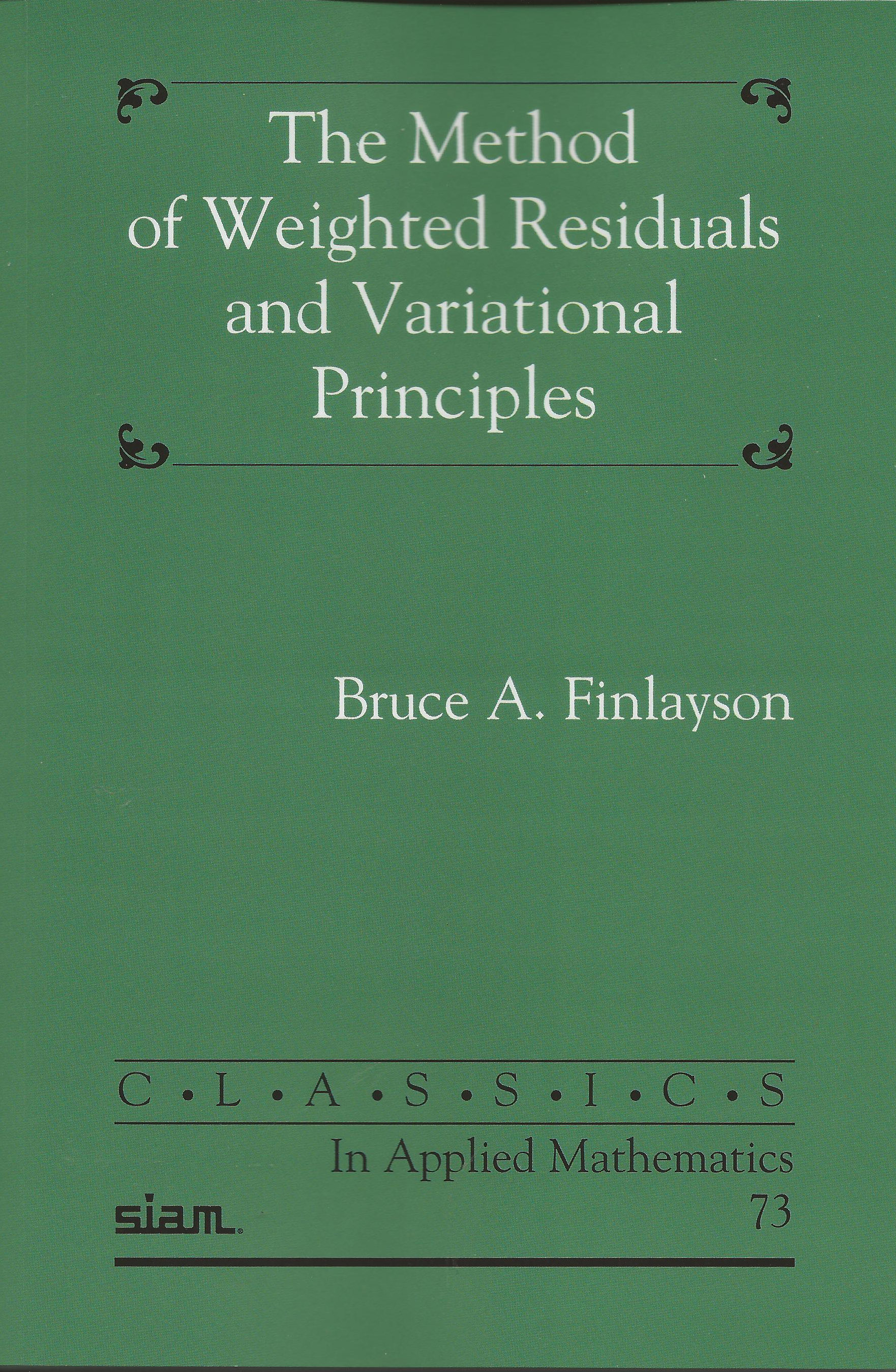• ## Method of Weighted Residuals and Variational Principles

####### What it covers

This book was originally published in 1972 when computers were just beginning to make an impact on the solution of differential equations. The first part of it treats differential equations governing transport problems, of flow, heat, and mass, using approximate methods and a series of functions, but using only a few terms. The Galerkin, collocation, least squares, and integral methods were all subsumed under the phrase Method of Weighted Residuals. The second part describes variational principles (or not) for those problems and shows how to find them and use them to construct error bounds and create stationary principles. Finally, the variational principles are used to derive error bounds that can be calculated for some problems. This was important because the series used in the approximate methods for nonlinear problems could not be extended to a large number of terms without the use of a computer. Since then computers have advanced considerably and much more sophisticated numerical techniques are available, but the mathematical principles described herein are still valid, and still useful.

#### Error Bounds

Chapter 11 in particular gives error bounds for many problems. Examples are given in the book, but there is a need to expand the coverage to include higher approximations and numerical methods. Thus, problems will be posted here (see right-hand side) that use the error bounds, but also use methods like the finite difference method and finite element method. Some of the problems are given with solutions (**), some with partial solutions (*), and some have no solutions on the website, but they are available to instructors via SIAM. These problems will be added as time permits, but they do give good examples of the value of error bounds and make use of modern computational tools like MATLAB®.

Undergraduate Research Projects at the University of Washington, 2001-2009

Introduction to Chemical Engineering Computing, Wiley (2012)